# Simple Formula for Driving Distance

Dave Tutelman -- December 27, 2014
This work was instigated by a couple of questions asked by Mike Stachura. He wanted to know how many yards of driving distance you get for every mile per hour of clubhead speed, and also how many extra yards of driving distance could a golfer pick up without increasing clubhead speed.
Reiterating Mike's two questions:
1. Is there a simple multiplier to relate driving distance to clubhead speed, something like "distance = 2.5 * speed"?
2. How much driving distance does the average golfer "leave on the table", assuming his/her clubhead speed is what it is and won't increase?
These are complementary questions, related by a paradox. Think about it; if there is a hard and fast answer to #1, then no distance is left on the table. If you know the golfer's clubhead speed, then you know the distance. So it is obviously more complicated than a simple formula that relates clubhead speed to distance. Here are some of the complications, and how we will deal with them to answer the questions:
• Ball flight is not linear, and there are no easy formulas to give an exact answer. To answer question #1, we are going to use the TrajectoWare Drive computer program, which deals explicitly with the complexity of ball flight. (If you want to see what makes the problem complex and how a computer deals with it, I have a tutorial on the subject.) We will see if we can make any easy generalizations from the computed results. Turns out we can.
• Because ball flight is complex, distance depends on a lot more than clubhead speed. To answer question #1, we will study the effects of clubhead speed, using the most reasonable or simple assumptions we can about the other things that affect distance. Then, when we get to question #2, we will hold the clubhead speed constant and open up the other assumptions, to see where we can do better.
• Mike wanted total driving distance, not just carry distance. This is a much harder problem to solve. I don't have a quantitative handle on bounce and roll after the ball lands ("runout"). But we do come up with some answers, based on research that others have done.
Let's dive into question #1: Is there a simple multiplier to relate driving distance to clubhead speed?

## Assumptions and approach

Mike was looking for a formula for driving distance based on clubhead speed. He wanted a simple formula of the type:

He started with the assumption that such a formula is possible. Here is his email to me, with the results of his research to that point.
At 11:01 AM 12/11/2014, Mike Stachura wrote:
I've studied a handful of trajectory programs out there, seen fitting charts companies have handed out to their fitters and tried to come up with a simple multiplier formula based on club head speed. Messing around with the numbers I came up with a series of stepped multipliers.
Less than 70 mph: 2.4
71-80: 2.45
81-90: 2.55
91-100: 2.65
More than 100: 2.7

But I'm afraid these yield distances that are too high for human golfers with real drivers. Maybe not?

I'll get back to the last conjecture -- about human golfers with real drivers -- but let's deal with these numbers first and what they ought to be. When you look at a simple fitting chart or a trajectory program, the author or programmer often assumed away much of the complexity. Let's see some of the typical assumptions:
• Clubhead speed is a given, it is fixed for this golfer.
• Center contact -- this implies two things:
• No gear effect.
• Maximum smash factor, 1.48 for low lofts.
• The 1.48 smash factor is itself based on another assumption: a modern ball and club with the maximum allowed coefficient of restitution of 0.83.
• Square clubface at impact; no sidespin.
• "Flat" attack, angle of attack=0.
• The loft at impact is that built into the clubhead. (Other things that can affect loft at impact are shaft bend and wrist cup.)
• Plain vanilla ambient conditions: temperature 80ºF, sea level, no wind, flat fairway, no elevation of tee box, etc.
• A properly fit driver. This is an important assumption! With all the other assumptions, the only fitting variable we have left is loft. The charts (and our study) will best fit the loft to the clubhead speed, consistent with all the other assumptions.
Let's make all these assumptions, plug them into the computer, and see what it gives us. Then we will try to find a simple formula that fits what is coming out of TrajectoWare Drive.

## Finding the formula

### Mike's multipliersBefore we turn to a program that really computes the ball flight, let's start with the multipliers Mike suggested. Five different multipliers for different clubhead speeds certainly isn't what you want; it's almost easier to remember the distances for each of those clubhead speeds. But let's look at the implications if that is the right "formula". Here is a graph of the distance implied by the varying multipliers. As we should expect, there is a discontinuity, or jump, every time the multiplier changes. The jumps range from 6 to 12 yards. So, for instance, the difference in distance between 89 and 90mph is 2.5 yards, but the difference between 90 and 91mph is 12 yards. This is not a helpful way to look at distance vs speed. So let's look to the actual, computed numbers and see what we get.

### Curves and lines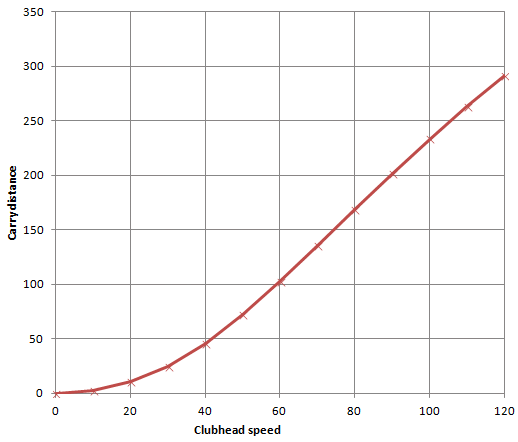Let's use the TrajectoWare Drive computer program to run the real ball flight, and plot the carry distance. We will do this for clubhead speeds from zero to 120mph. For each clubhead speed, we will find the driver loft that gives the best carry distance for that clubhead speed. (TrajectoWare drive makes this easy; you can use the scroll wheel on the mouse to vary loft, and watch the carry distance number for a maximum.)

Here is the graph I got.

.
Let's take this plot, and try for multiplier's like Mike's for 60mph, 70mph, 80mph, 90mph, and 100mph. We get:

 Clubhead speed Carry distance Multiplier 60 103 1.71 70 136 1.94 80 169 2.11 90 202 2.24 100 233 2.33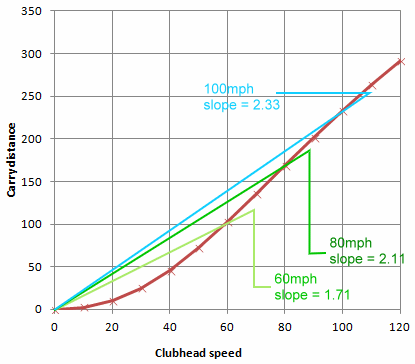A few observations:
• The most obvious is that the multipliers are lower than the ones Mike suggested. Upon further questioning, I found out he is looking at total distance rather than carry distance. So you would expect his multipliers to give longer distance. We will revisit this below.
• Apart from that, the trend is similar to Mike's multipliers. We don't have one single multiplier. Instead, we have different multipliers for different clubhead speeds, and they increase with clubhead speed. So they are no more useful than Mike's multipliers.
One way to view the multipliers is shown on this graph, derived from the graph above. I have drawn straight lines from the origin [0,0] to the actual distance at three speeds: 60mph, 80mph, and 100mph. The slope of each straight line is the multiplier for that distance. Looking at the graph, we can see why there is a different multiplier for each clubhead speed; the graph (the red curve) is not a straight line. In order for there to be a simple formula of the form distance=speed*multiplier, the graph would have to be a straight line through the origin!

It isn't. We can tell just by looking. Does that mean a simple formula is a hopeless dream? No! Looking at the graph, we can see something very interesting that will help us simplify the problem.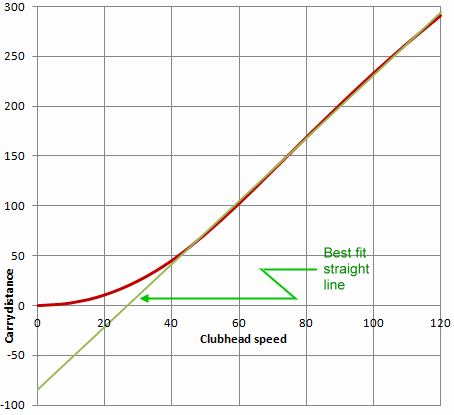There is indeed a section of graph that looks like a straight line. It is the part from 60mph to 120mph.

I use Excel for plotting the graphs, and I can ask it to do curve fitting to the function I plot. I asked for the best-fit straight line to the straight-looking section of the red curve (the actual distances). Excel gave me the green line on the graph, and told me that the equation for that line is:
Distance = 3.16*speed - 85.2

Let's look at this line and see what the differences are between this and what Mike -- and most people who look for a simple formula -- assume:
• It is not just a multiplier; there is a multiplication and an addition. (Well, a subtraction, but that is addition of a negative number.)
• The line does not go through the origin. At zero mph of clubhead speed, the ball goes 85 yards backwards. That's crazy.
Well, it would be crazy if we were crazy enough to try to use it at 0mph clubhead speed. But remember where this line comes from. It is a best fit to the curve over the range from 60mph to 120mph. We really should only use it within this range. (Actually, it is just as good at 50mph, then it ceases to be a good estimator.)

How good is the match between the straight line and the red distance curve? Let's look at two different ways to measure it.
1. When Excel plots a best-fit line, it gives a measure of fit called "R-squared". When R-squared is 1.0, that is a perfect fit to the data. When R-squared is zero, there is no correlation at all between the data and the line we have claimed fits it. In the case of our carry distance curve, R-squared is 0.9991. "Three nines" means the line is an extremely good fit to the data.
2. I have compared the data to the line at 10mph intervals. I found agreement within two yards from 50mph to 110mph. It was up to a three-yard difference at 120mph, still quite good.

## Explanations and variations

### What is going on, and why?

So we have a very effective, simple formula, but not what Mike expected. Why not? Because it isn't based on just a multiplier, a proportion between clubhead speed and distance. But we have found a linear relationship between carry distance and clubhead speed, that stays linear over the range of interest. 60mph-120mph covers the vast majority of all golfers.

If we insist on a single multiplier to do the job, we will be disappointed. But we learned in high school math that the formula for a straight line is:
y = mx + b
where:
m is the multiplier (the slope of the line),
b is the y value where x=0, the place where the line intersects the y-axis, what is called the "y-intercept".

Insisting on a single-multiplier solution would add the restriction that b=0, and that prevents the solution from being very good. By allowing a non-zero y-intercept, we get a very good linear approximation, which is also a very simple formula for distance. True, you have to remember two numbers, a multiplier and an intercept. But that is better than needing to remember five different multipliers, along with the speed range to which each applies. And more accurate, I might add.

### Ball speed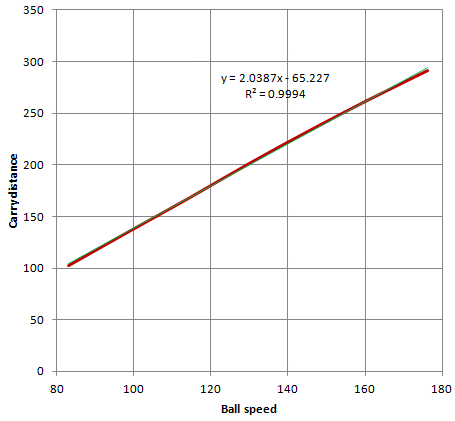While we're getting a simple linear relationship for clubhead speed and distance, what about a similar relationship for ball speed and distance. That way, we don't have to assume perfect contact, a maximum smash factor. We can compute ball speed using whatever smash factor the golfer is able to generate, then go from ball speed to distance. To do this, I recorded the ball speed when I did the Excel graphs for clubhead speed vs distance. Here is a plot of ball speed vs distance, and its best-fit straight line. Points worth noting: It is hard to visually separate the red data curve from the green best-fit line. That is supported by the R-squared of 0.9994, three-and-a-half nines. The slope is very close to 2.0. For all practical purposes, we can say that every 1mph increase in ball speed causes a 2yd gain of carry distance. Again, there is a y-intercept. The straight line does not go through zero. Thus it will give nonsense if we try to use it for very small ball speeds. But in the range of interest, ball speeds produced by swings between 60 and 120mph, it gives very good estimates.

### How do we use the formula?

Two ways, depending on what you are trying to accomplish:
1. The obvious one: given a clubhead speed, what is a reasonable distance you can expect to hit it? Here's how you do that:
• Multiply the clubhead speed by 3.16.
• Subtract 85.2 yards from the result.
• You have the carry distance, within one or two yards.
2. You can do something that will gain a few MPH of clubhead speed. (Clubfitters work problems like that a lot.) How many yards is that worth?
• Multiply the gain of speed by 3.16. Actually 3 is close enough for most such problems. Not many things you can do to gain more than 6mph, which is the minimum before you can see much difference between 3 and 3.16.
• You have the gain in carry distance.
If you are given ball speed instead of clubhead speed, the numbers are:
1. Multiply by 2.04 and subtract 65.2 yards, for the first case.
2. Multiply by 2 for the second case.

### Can we make it even simpler?

No we can't, at least not without increasing the error. But, if you're OK with a 5yd error at the extremes, and less than a 3yd error between 70mph and 108mph, you can round things off to:

CarryDistance = 3 * ClubheadSpeed - 71

That is really simple.

## Total distance

Mike's question was really aimed at total distance. TrajectoWare Drive gives carry distance, not total distance. Total distance is difficult to compute in general. Making it harder is the fact that it depends on the fairway conditions where the ball lands. Baked fairways prepared for PGA Tour events give very different runout from the soft courses that I am playing in New Jersey in the late fall. And also different from the hard-frozen fairways (more resembling concrete) that friends Bill, Jim, and I expect to find tomorrow at our traditional New Years Eve round.

But there is some hope. There are people doing studies of total distance, and apparently making progress. And we do have a little data from those studies...

### Revisit Mike's multipliers

I asked Mike if the multipliers he cited are for carry distance or total distance. He cited his earlier email, and clarified it with another email.So Mike has already digested a handful of studies of total distance. Let's look again at that graph of the multipliers he came up with, but this time we will ask Excel to show us the best-fit straight line. The line is very interesting for a number of reasons:
• The R-squared value says it is quite a good match to the data, even if the data is a little jagged. It is visibly not as good as the carry distance match, but "two nines" is not bad at all.
• The slope is the same as the slope of the carry distance line. This one is 3.1604, and the carry distance is 3.1632. That is more exact than you would ever expect. So...
Let's compare the simple equations for carry distance Dc and total distance Dt.

Dc = 3.16*speed - 85.2
Dt = 3.16*speed - 50.5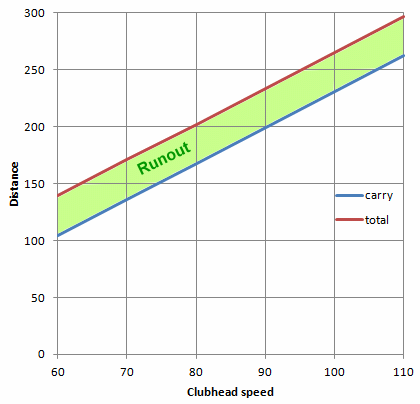What this says is that the runout is 35 yards, no matter what the clubhead speed. Here's a graph to show what it looks like.

That is not only interesting, it is suspect. Might be true, but it defies intuition -- at least it defies my intuition. I would think that a drive struck at 100mph will bounce and roll out farther than one struck at 70mph. I need more data and a deeper understanding before this makes sense.

Let's look for other data to confirm or deny this curious result.

### TrackMan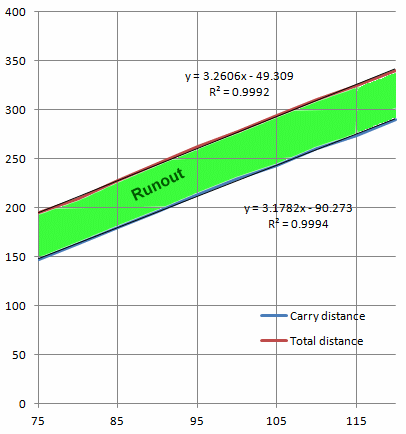TrackMan has published a Total Distance Driver Optimization chart that I have seen numerous places on the Internet. It gives the impact and launch conditions for maximum total distance, for a variety of clubhead speeds between 75mph and 120mph. It also lists both carry and total distance. So we have enough information to make a graph comparable to the previous one.

Like our other results, the carry and total distances are straight-line graphs (to three nines and better) over the range of interest. And, like the graph we interpolated from Mikes multipliers, the runout seems not to depend on clubhead speed. (Well, it does -- a little. At lower speeds the runout is about 48 yards; it climbs to 50-51 at higher speeds. Still, almost constant runout, regardless of clubhead speed.)

I conclude a few things from this exercise:
• The runout is approximately the same -- about 50 yards -- for all clubhead speeds, if the driver loft was optimized for that speed.
• Justin Padjen of TrackMan tells me that the data for their runout model was gathered from PGA Tour events, where the fairways are dry and hard. So there is more runout than the average golfer would experience. We should probably keep our total distance formula based on the composite model from Mike Stachura's data.
Something to keep in mind about the runout being independent of clubhead speed. What we have seen so far is data for distance-optimized drivers with very specific constraints. If you are using a club not distance-optimized for the clubhead speed, or optimized for different constraints (like non-zero attack angle) or you strike it badly, you are likely to get a very different runout. So don't generalize this beyond the constraints that apply here.
By the way, I did a "sanity test" -- a consistency check -- to be sure that TrackMan and TrajectoWare Drive were generating comparable answers. The TrackMan optimization charts had full launch conditions for each clubhead speed: ball speed, launch angle, and spin. I plugged these into TrajectoWare Drive to find the carry distance. In every case, the carry distance for TrajectoWare Drive and TrackMan were less than 3 yards apart. So the numbers we see are quite comparable.

### Cochran & Stobbs - 1968

I found further confirmation in an interesting and unexpected place.

Return with us now to those thrilling days of yesteryear! In 1968, Alastair Cochran and John Stobbs wrote what I think is still the best book around for getting started in golf science. "The Search for the Perfect Swing" is a genuine classic.

I'm the sort of person who reads appendices, and Appendix I includes equations for carry distance and total distance. And lo and behold, they are linear equations of the form y=mx+b. To get them into a form we can compare, I had to:
• Convert the speed units from feet/sec to mph. That's a factor of 1.47.
• Convert ball speed (the C&S equations were in ball speed) to clubhead speed. But remember, this was 1968. No spring face drivers, just wooden heads. No "rabbit ball". The COR cited in the book was only 0.67. That gives a maximum smash factor of only 1.36.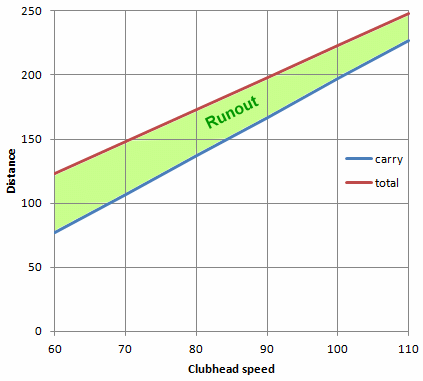The converted equations, shown here in a graph, were:

Dc = 3*speed - 103
Dt = 2.5*speed - 27

Much to my surprise, the runout actually decreased with clubhead speed. So maybe a constant runout isn't far-fetched after all. If the C&S equations were correct for the day (and I have to believe they were), then the question to ask is, "What has changed since 1968 to improve runout for big hitters more than for shorter hitters?"

The thing that comes to mind immediately is the modern tournament ball. In the past 10-15 years, ball manufacturers have managed to make balls with less tendency to spin as they compress more on the clubface. Not that they spin less -- higher clubhead speed will always generate more spin -- but they acquire spin at a lower rate as they compress more.  A lower spin means (a) less runout-killing backspin on landing, and (b) a less steep angle of descent. (AoD is as harmful as backspin to runout.)

So, to my surprise, it is conceivable and even likely that today the runout does not vary with clubhead speed. The additional velocity on landing is countered by spin-related problems: angle of descent and backspin on landing. The data in these last two graphs suggests that it took big changes in modern ball design (think Pro-V1x and comparable balls) to get the big hitters' runout to even equal the mere mortals' with a driver.

## Conclusion

There is a good answer to the first of Mike's questions, but it is not in the form he assumed. It does not consist of a single multiplier, but rather a straight line that does not pass through the origin. The formula is

Carry distance = 3.16*speed - 85.2

But Mike wanted total distance, not just carry distance, and his data is probably the most applicable to the typcial golfer. The best-fit straight line to the Mike's data is

Total distance = 3.16*speed - 50.5

Note that the carry distance formula above relates to optimizing carry distance. The carry distance you realize when you optimize total difference will be somewhat lower.

On to Mike's second question: how much can typical golfer improve his or her distance, without having to achieve more clubhead speed.

Last updated - Jan 12, 2015